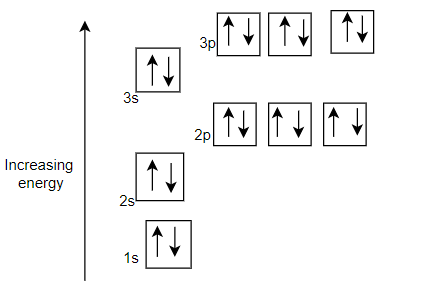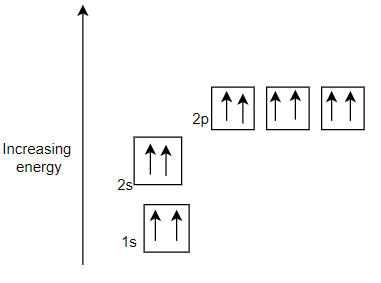# Pauli exclusion Principle

## Pauli exclusion Principle

• This principle was given by the Austrian scientist Wolfgang Pauli (1926)
• The number of electrons to be filled in various orbitals is restricted by the exclusion principle
• According to this principle no two electrons can have same set of 4 quantum number
• As per this rule,it is impossible for two electrons of a poly-electron atom to have the same values of the four quantum numbers: n, the principal quantum number, l, the azimuthal quantum number, $m_l$, the magnetic quantum number, and $m_s$, the spin quantum number. For example, if two electrons reside in the same orbital, then their n, l, and $m_l$ values are the same, therefore their $m_s$ must be different, and thus the electrons must have opposite half-integer spin projections of 1/2 and -1/2.
• It can be also stated as only two electron can exists in the orbital and these electrons must have opposite spin
• for n=1
1s subshell can have maximum 2 electrons
Total Number of electron= 2
for n=2,
1s subshell can have maximum 2 electrons
p -subshell can have maximum 6 electrons
Total Number of electron= 8
for n=3
3s subshell can have maximum 2 electrons
3p -subshell can have maximum 6 electrons
3d- subshell can have maximum 10 electrons
Total Number of electron= 18
This can be summed up as : the maximum number of electrons in the shell with principal quantum number n is equal to $2n^2$.
• For the two electron in the orbital, the first three quantum number(n,l,$m_l$) are same, only the spin quantum number ($m_s$) differ. One electron will have up-spin ( +1/2), the other must be down-spin (-1/2)
• Here is the correct configuration• Here is the incorrect configurationQuestion 1
Pauli's exclusion principle states that
(a) Nucleus of an atom contains no negative charge
(b) Electrons move in circular orbits around the nucleus
(c) Electrons occupy orbitals of lowest energy
(d) All the four quantum numbers of two electrons in an atom cannot be equal
Solution
(d)

Question 2
The electrons would go to lower energy levels first and then to higher energy levels according to which of the following
(a) Aufbau principle
(b) Pauli's exclusion principle
(c) Hund's rule of maximum multiplicity
(d) Heisenberg's uncertainty principle
Solution
(a)

Question 3
The number of electrons which can be accommodated in an orbital is
(a) One
(b) Two
(c) Three
(d) Four
Solution
(b)

Question 4
The maximum number of electrons that can be accommodated in 'f' sub shell is
(a) 2
(b) 8
(c) 32
(d) 14
Solution
(d)